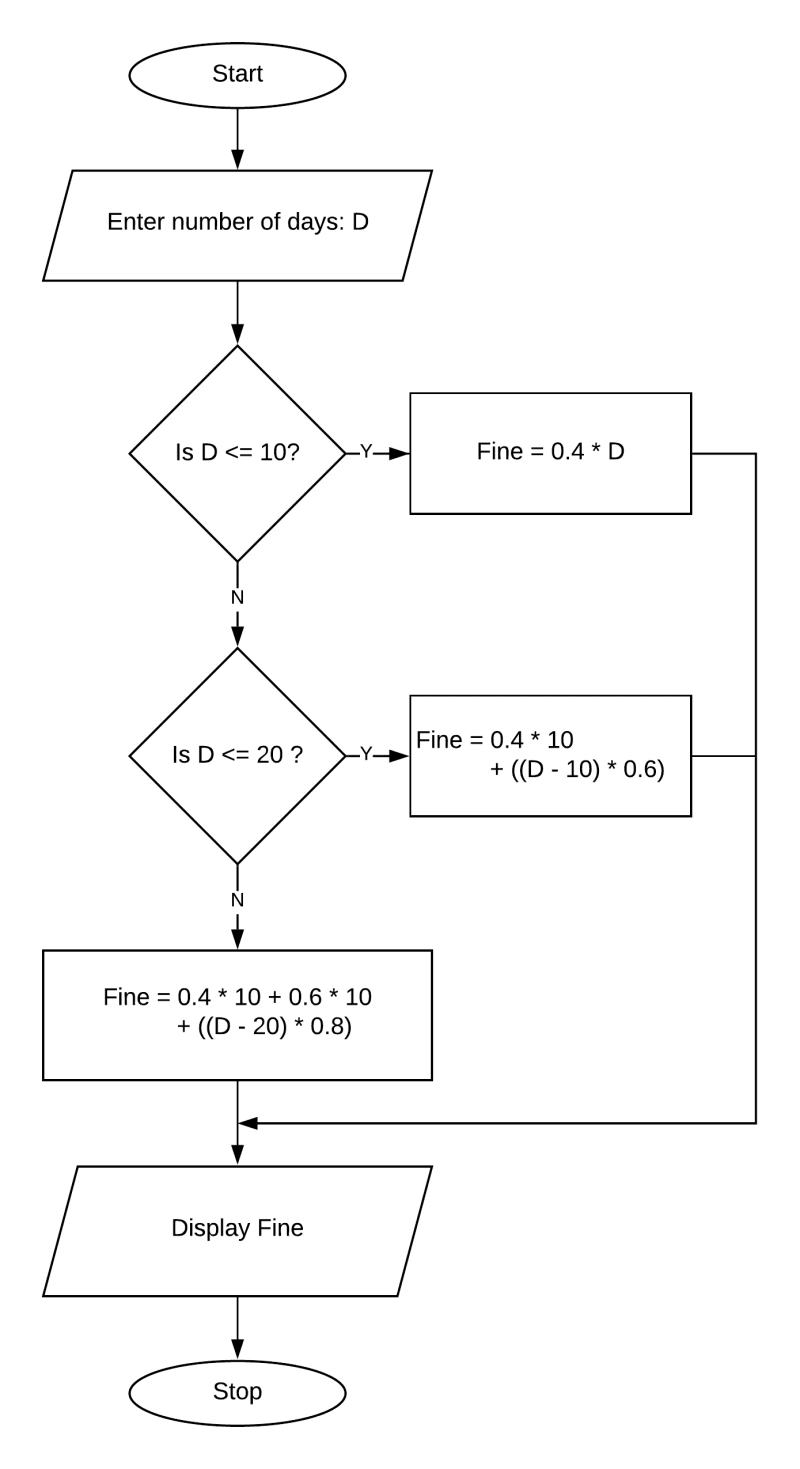# Algorithms and Flowcharts

## Choose the correct option

#### Question 1

Who invented the algorithm?

1. Charles Babbage
2. Md. Musa Al Khwarizmi
3. Blaise Pascal

Md. Musa Al Khwarizmi

Reason — The origin of algorithm is attributed to the Persian astronomer and mathematician, Abdullah Mohammad bin Musa Al Khwarizmi.

#### Question 2

Who among the following is the first to write algorithm used for computer operation?

2. Charles Babbage
3. Alan Turing

Reason — Lady Ada Lovelace was the first person who created the first algorithm that could be executed on a computer.

#### Question 3

Which of the following is used as the beginning step in an algorithm?

1. Get
2. Set
3. Start
4. Stop

Start

Reason — Start is used as the beginning step in an algorithm.

#### Question 4

Which of the following symbols is used to start a flowchart?

1. Oval
2. Terminal
3. Connect
4. Rhombus

Terminal

Reason — A terminal box with the word 'START' is used at the beginning of the flowchart.

#### Question 5

Which of the following boxes is used for displaying an output statement?

1. Rectangular box
2. Rhombus box
3. Parallelogram box
4. Oval box

Parallelogram box

Reason — The output statement is represented by a parallelogram.

## Fill in the blanks

#### Question 1

The first symbol in a flowchart is the START symbol.

#### Question 2

A flowchart is a pictorial representation of a sequence of instructions that are required to solve a problem.

#### Question 3

Flow lines are used to connect different boxes.

#### Question 4

The Decision Box is used for making decisions as well as comparisons.

#### Question 5

All mathematical operations are put in a Process box.

## Write the names & the symbols

#### Question 1

Write the names and the symbols of the boxes for the given statements

StatementName of the BoxSymbol
To assign a number
To display the result
To start the process
To decide if a number is smaller than the other

## Complete the following flowcharts

#### Question 1

A flowchart to check whether a triangle is possible or not.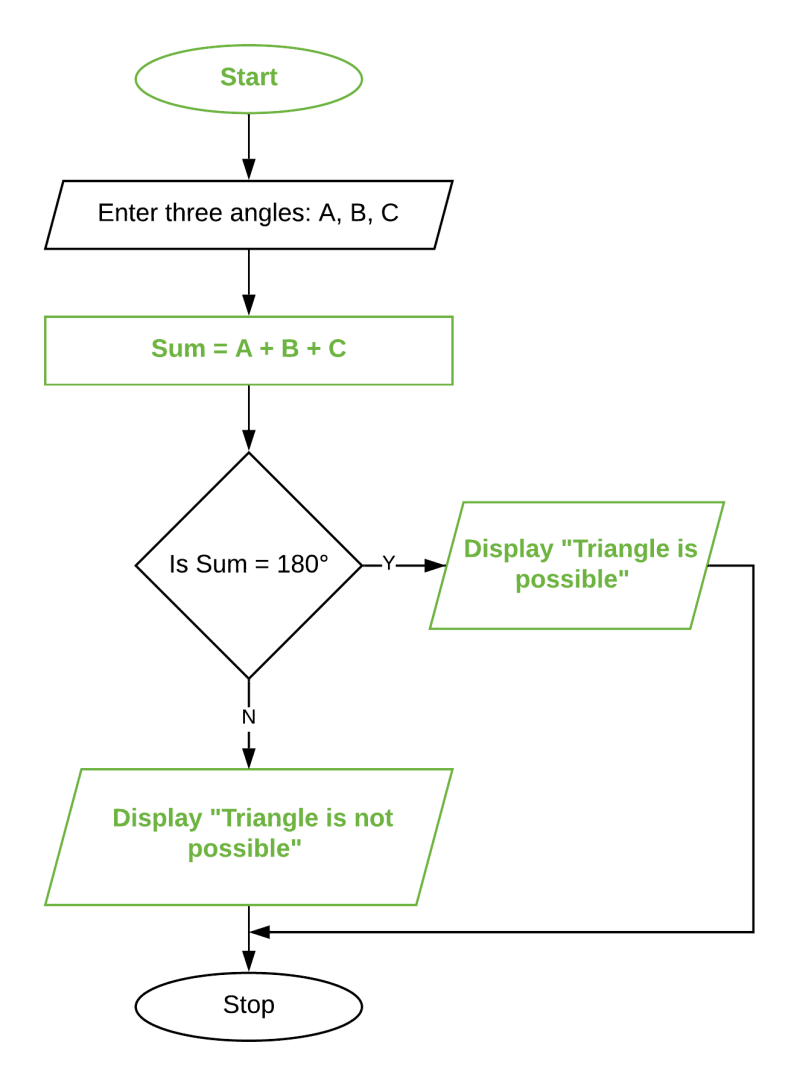#### Question 2

A flowchart to display the greater of the two numbers.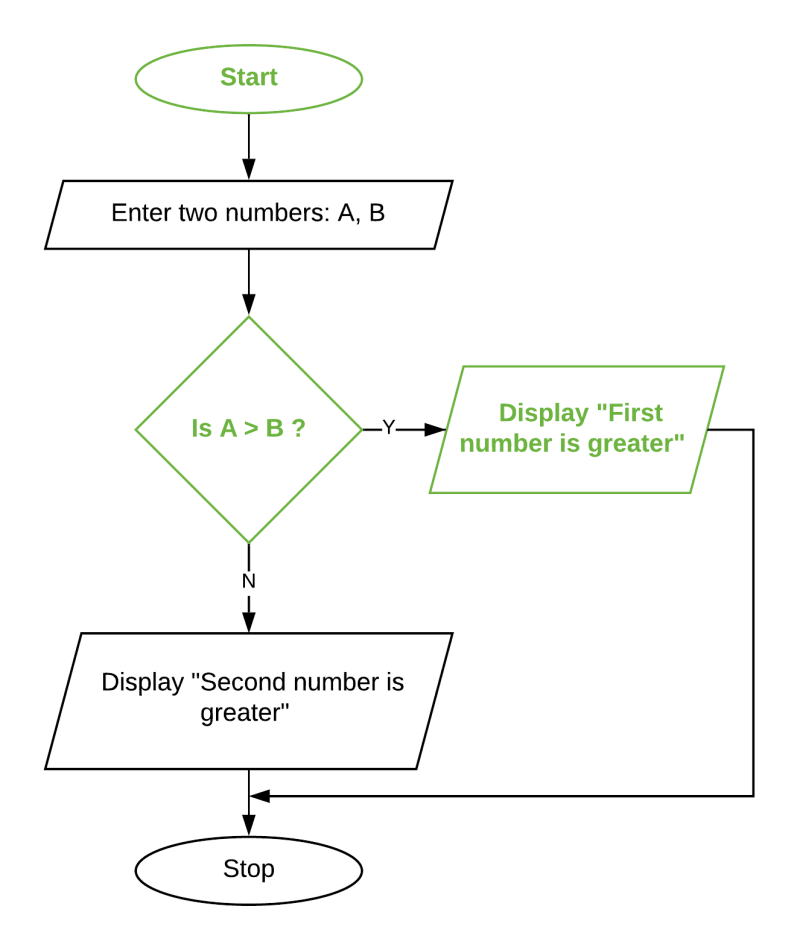## Case-Study Based Questions

#### Question 1

As a homework assignment, your teacher has given a flowchart (shown below) to find and display the square and cube of a positive number. The execution must be terminated, if a negative number is entered.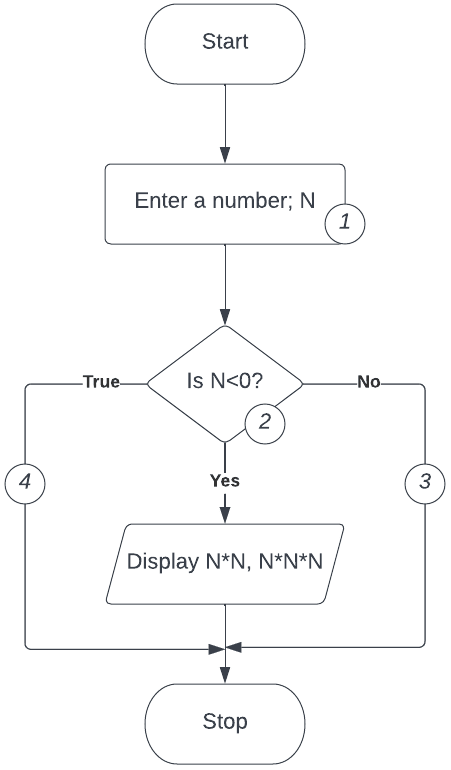The above flowchart has some errors which are indicated with (1), (2), (3) and (4). Answer the following questions to give the correct result.

(a) What will be the correct representation of the box in (1)?

(b) What will be the correct logic for the variable N in (2)?

(c) Out of the flow lines (3) and (4), which one is undesirable?

(d) When all the corrections are made, what will be the output, if the input value is taken as 12?

(a) The correct representation of the box in (1) is a parallelogram or input/output box.

(b) The logic for the variable N in (2) will be - If N>0?

(c) Flow line (4) is undesirable.

(d) The output will be 144, 1728.

The corrected flowchart is shown below: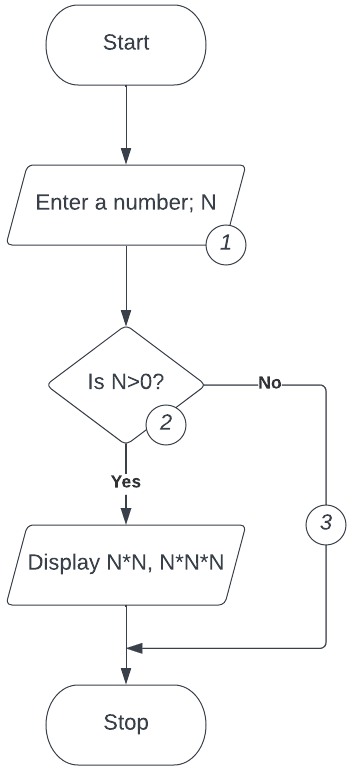#### Question 2

Anuj, a class 9 student, has designed the following flowchart to input a positive number and check and display whether the number is a single digit number, a double digit number or a triple digit number. To assess his brother who is in class 8, he hides four statements in the flowchart.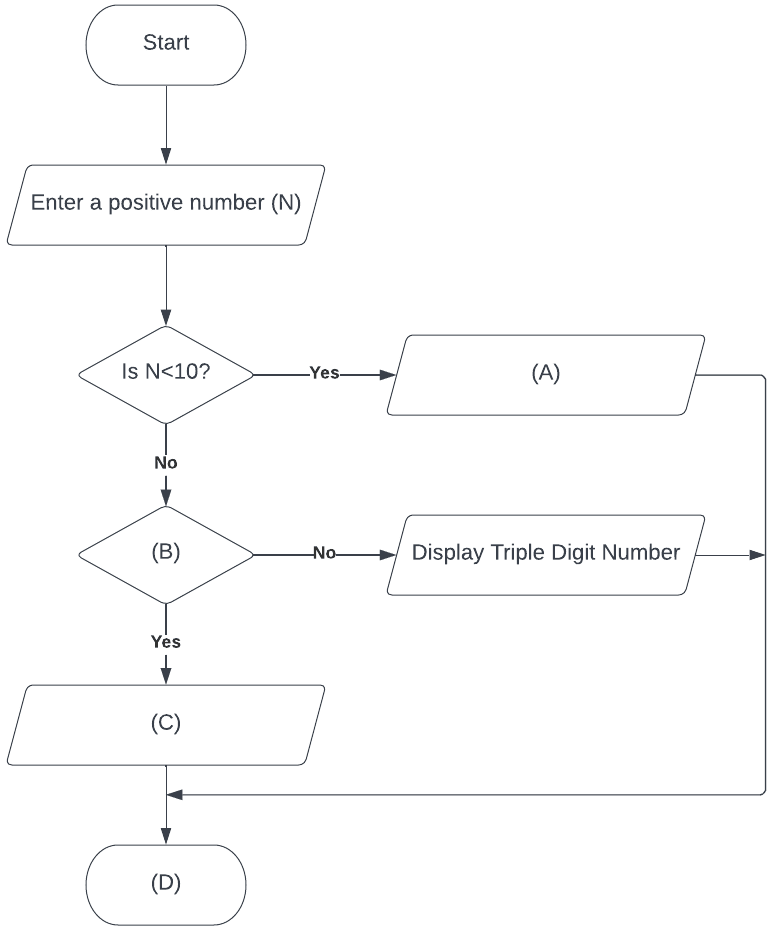Based on the above case and the given flowchart, answer the following questions:

(a) Which of the following statements will be filled in place of (A)?

1. Display Single digit number
2. Display Double digit number
3. Display Negative number
4. Display odd number

(b) Identify (B) from the following:

1. N >= 100
2. N <= 100
3. N < 100
4. N > 100

(c) Which of the following statements will be filled in place of (C)?

1. Display Single digit number
2. Display Double digit number
3. Display Negative number
4. Display odd number

(d) Identify (D) from the following:

1. Start
2. End
3. Finish
4. Stop

(a) Display Single digit number

(b) N < 100

(c) Display Double digit number

(d) Stop

The complete flowchart is shown below: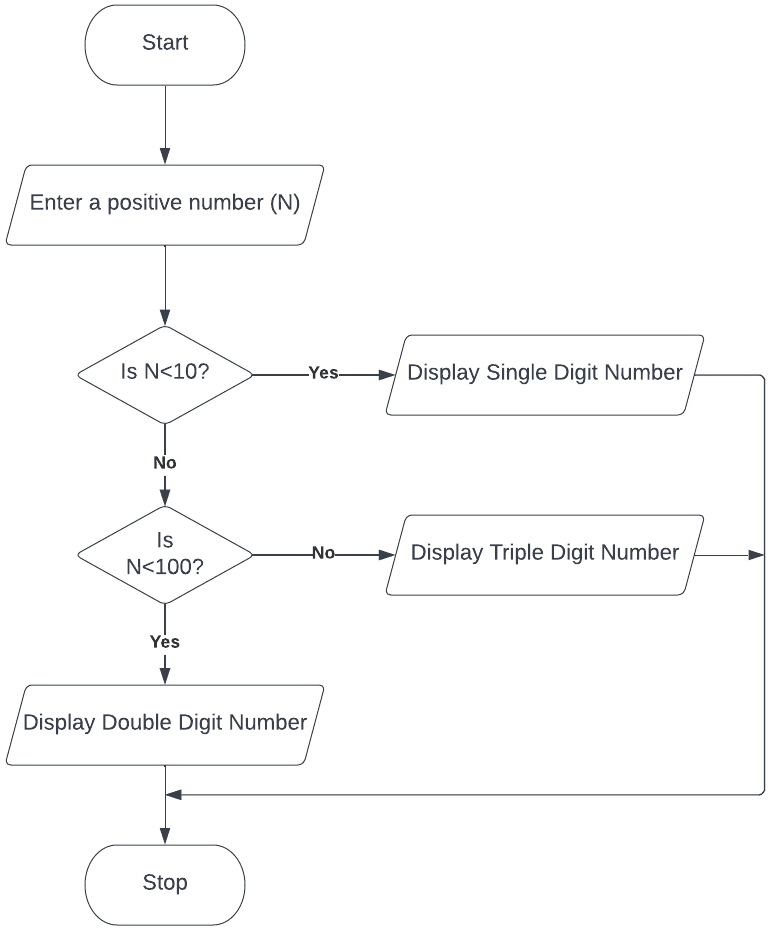## Write algorithms for the following

#### Question 1

To go for a class picnic

Step 1: Start
Step 2: Decide the picnic venue, date and time
Step 3: Decide the picnic activities
Step 4: Hire a vehicle to reach to the venue and comeback
Step 5: Go to the picnic venue on the decided date
Step 6: Do the activities planned for the picnic
Step 7: Come back to school in the hired vehicle
Step 8: Stop

#### Question 2

To celebrate New Year

Step 1: Start
Step 2: Prepare a guest list for New Year party
Step 3: Decide the venue, food menu, games and fun activities for the party
Step 4: Invite the guests for the party
Step 5: On New Year eve, get ready and enjoy the party
Step 6: Stop

#### Question 3

To make tea/coffee

Step 1: Start
Step 2: Boil water in a saucepan
Step 3: Add tea to boiling water
Step 4: Add sugar to boiling water
Step 5: Add milk to boiling water
Step 6: Boil this water with all the ingredients for 2 mins
Step 7: Sieve the tea in a cup
Step 8: Stop

#### Question 4

To celebrate Teachers' Day

Step 1: Start
Step 2: Decide the activities for teachers' day like dance performances, plays, etc
Step 3: Form groups of students and assign the decided activities from step 2 to each group
Step 4: Decide the practice timings for each group
Step 5: Each group to practice as per the timings decided in step 4
Step 6: Invite the teachers to Teachers' Day celebrations
Step 7: Perform the activities planned in step 2 on Teachers' Day
Step 8: Stop

#### Question 1

What is a flowchart? What are the features of a flowchart?

A flowchart is a pictorial representation of various steps involved in a process. It is used to depict flow of an algorithm. It is constructed with the help of boxes and arrows (flow lines). As a flowchart depicts the flow of operations, any error in logic can be easily detected.

The features of a flowchart are as follows:

1. It is an easy method of communication.
2. It is independent of any programming language.
3. It is the key to correct and accurate programming.
4. It helps to plan and design a new system.
5. It clearly indicates the task to be performed at each level.

#### Question 2

What is an algorithm? What are its characteristics?

An algorithm is defined as a sequence of instructions written in a step by step manner to get the desired results. It helps to develop the fundamental logic of a problem and is an effective method to solve different problems.

Some characteristics of an algorithm are as follows:

1. Each step of an algorithm must be precisely defined.
2. An algorithm must contain blocks that will help to solve problems more efficiently and logically.
3. It should accept a set of inputs and produce a defined output.
4. It must terminate after a finite number of steps.
5. It should be independent of any computer programming language.

#### Question 3

Define the following and draw their symbols:

(a) Process Box

A process box is used to represent all types of mathematical operations and assignment of values. It is rectangular in shape. Thus, arithmetic operations like addition, subtraction, multiplication and division can be shown in this box.(b) Terminal Box

This box is used to indicate the beginning (START) and the end (STOP) of a process. It is an oval or a rounded rectangle. A terminal box with the word 'START' is used at the beginning of the flowchart and a box with the word 'STOP' is used at the end of the flowchart.(c) Input/Output Box

These boxes are used to represent the input/output operations of a process. The I/O box is represented by a parallelogram. All the commands required to be taken or given are put into these boxes. Thus, statements like INPUT, PRINT etc., are used in these boxes.(d) Decision Box

The decision box is used to represent the statements which can either result in a True/False (Yes/No) condition. It is in a shape of a diamond or a rhombus. If the statement results in True (Yes) then the statements following the 'true' condition are implemented, otherwise the statements following the 'false' condition are implemented.## Write algorithms & draw flowcharts

#### Question 1

Accept the length of two different line segments and check whether they are equal or unequal. Display the message accordingly.

Algorithm

Step 1: Start
Step 2: Accept the length of the two line segments as l1 and l2.
Step 3: If l1 and l2 are equal, then display 'Line Segments are equal'.
Step 4: If l1 and l2 are not equal, then display 'Line Segments are not equal'.
Step 5: Stop

Flowchart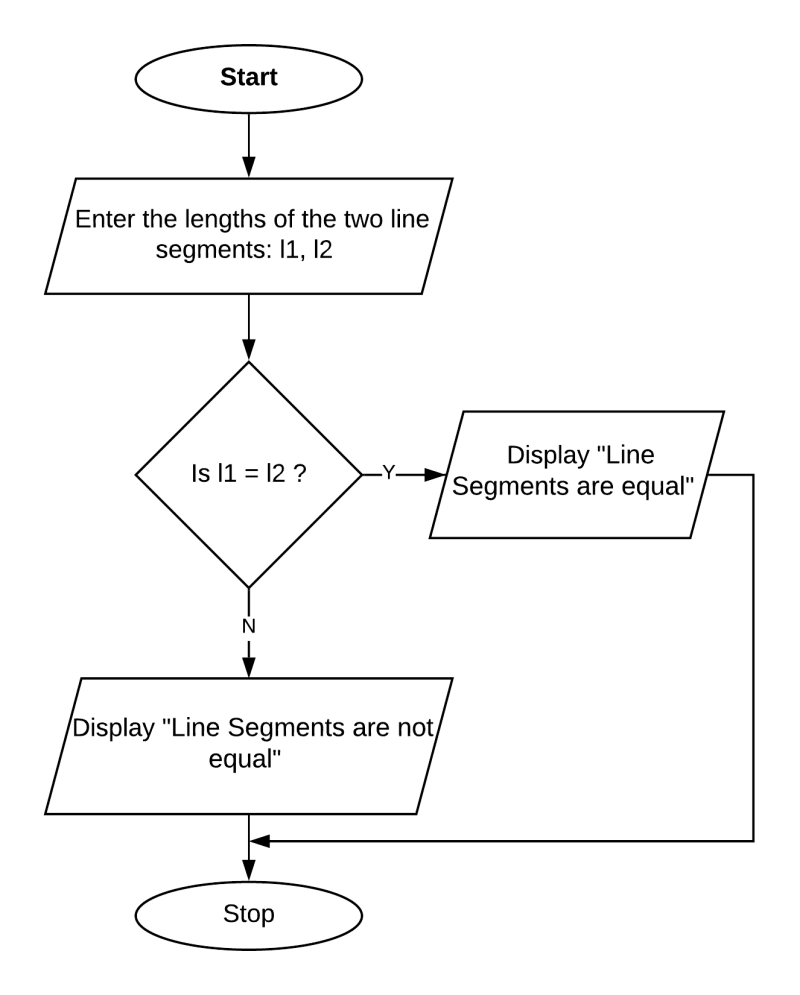#### Question 2

Accept the age of a person and check whether he/she is eligible to vote or not. A person is eligible to vote only when he/she is 18 years or more.

Algorithm

Step 1: Start
Step 2: Accept the age of the person.
Step 3: If age is greater than or equal to 18, then display 'You are eligible to vote'.
Step 4: If age is less than 18, then display 'You are not eligible to vote'.
Step 5: Stop

Flowchart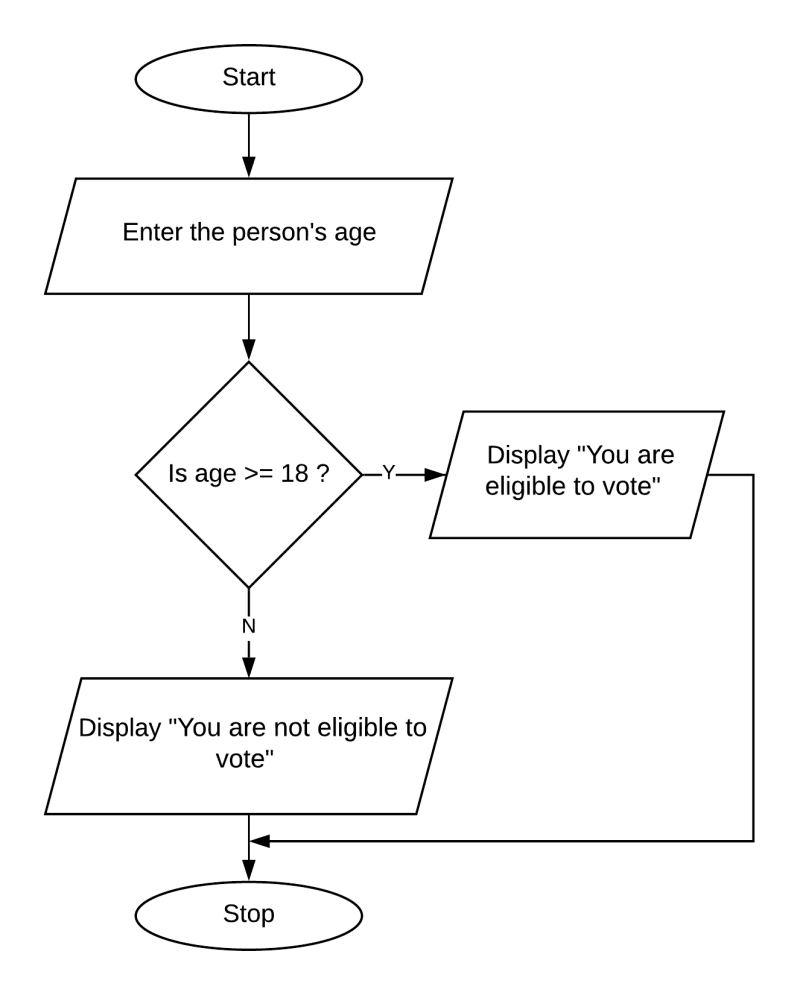#### Question 3

Accept three numbers and check whether they are 'Pythagorean Triplets' or not. Display the message accordingly.
(Hint: Use Pythagoras Formula for a Right-angled Triangle: h = p2+b2)

Algorithm

Step 1: Start
Step 2: Take 3 numbers as input (Say A,B,C)
Step 3: If A2 = B2 + C2, display 'Pythagorean Triplets'
Step 4: If B2 = A2 + C2, display 'Pythagorean Triplets'
Step 5: If C2 = A2 + B2, display 'Pythagorean Triplets'
Step 6: If no condition of Step 3,4,5 match, display 'Not Pythagorean Triplets'
Step 7: Stop

Flowchart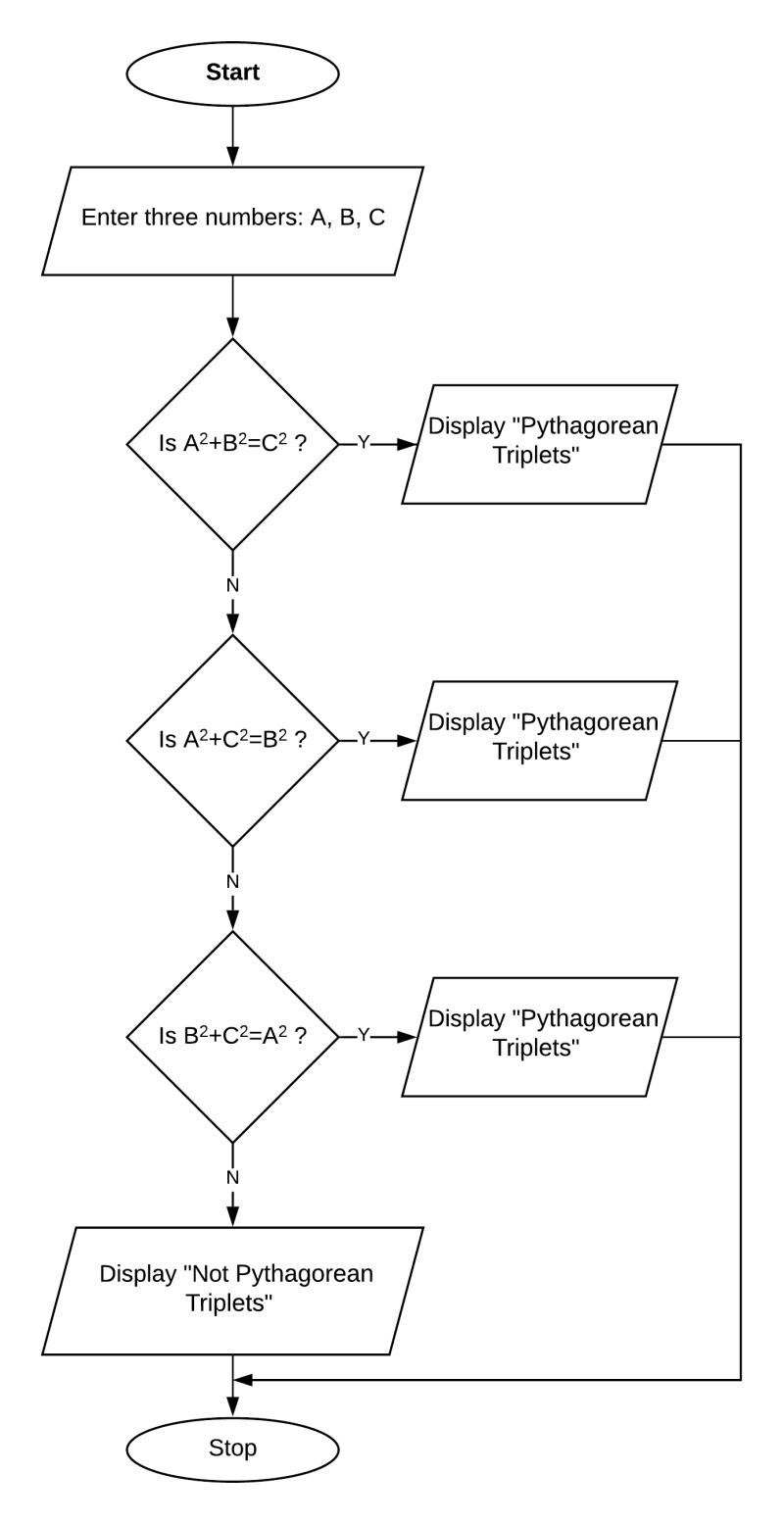## Draw flowcharts for the following

#### Question 1

In a cricket match, a viewer carries a laptop in the stadium every day to keep record of the runs made by each batsman. When a player comes to bat, the viewer enters the previous runs made by the player. Further, he enters the runs scored for each ball. When the runs entered on a ball is -1, it means the player is out. The laptop then displays the runs made by the player in the current innings as well as the total. The process continues until all the players are out. Draw a flowchart for the task stated above.

Flowchart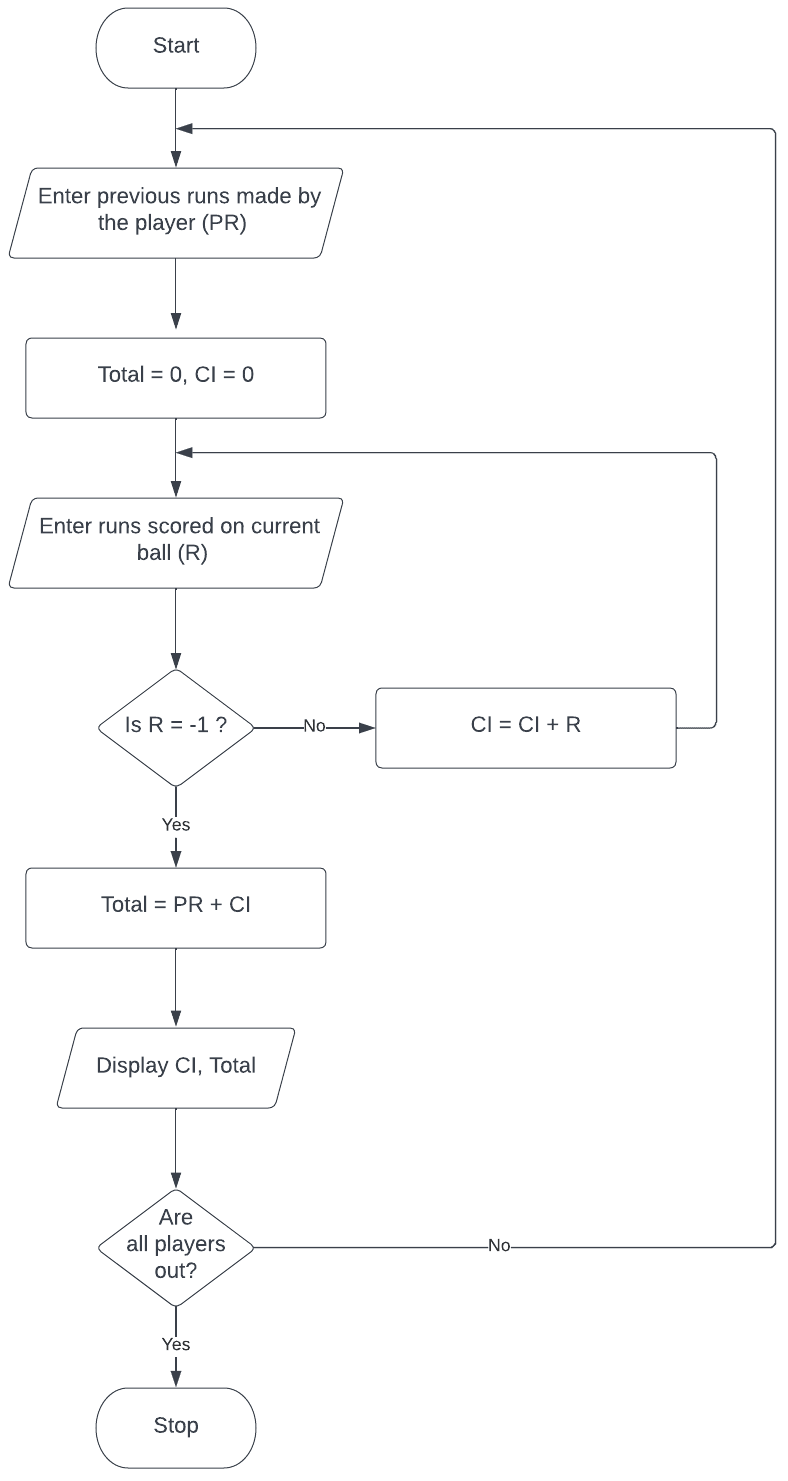#### Question 2

Accept a number and check:

(a) whether the number is divisible by 2 and 5.

(b) whether the number is divisible by 2 but not by 5.

(c) whether the number is divisible by 5 but not by 2.

Display the message accordingly.

Flowchart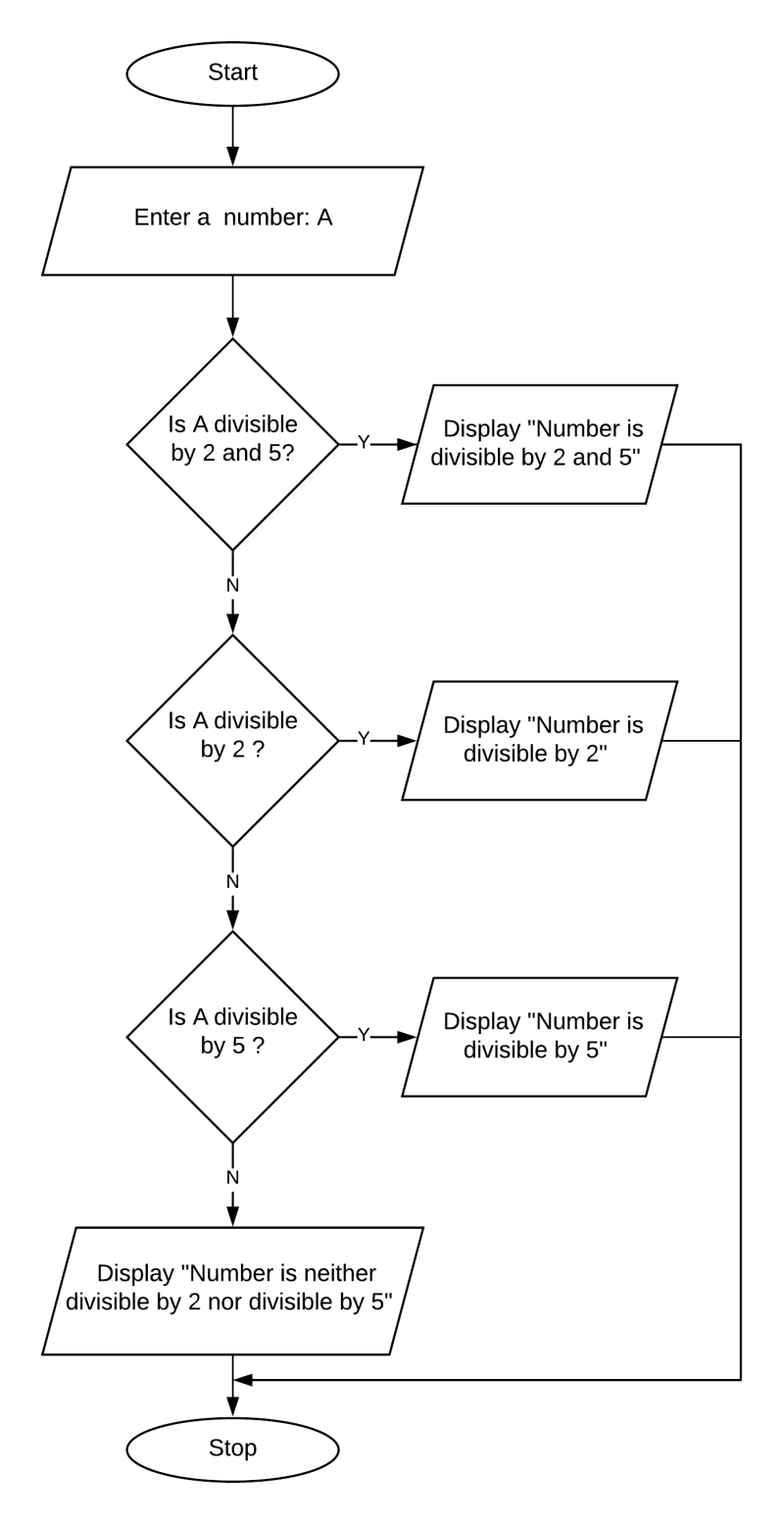#### Question 3

Input three angles of a triangle and check whether a triangle is possible or not. If possible, then check whether it is an 'Acute-angled Triangle', 'Obtuse-angled Triangle' or a 'Right-angled Triangle'.

Flowchart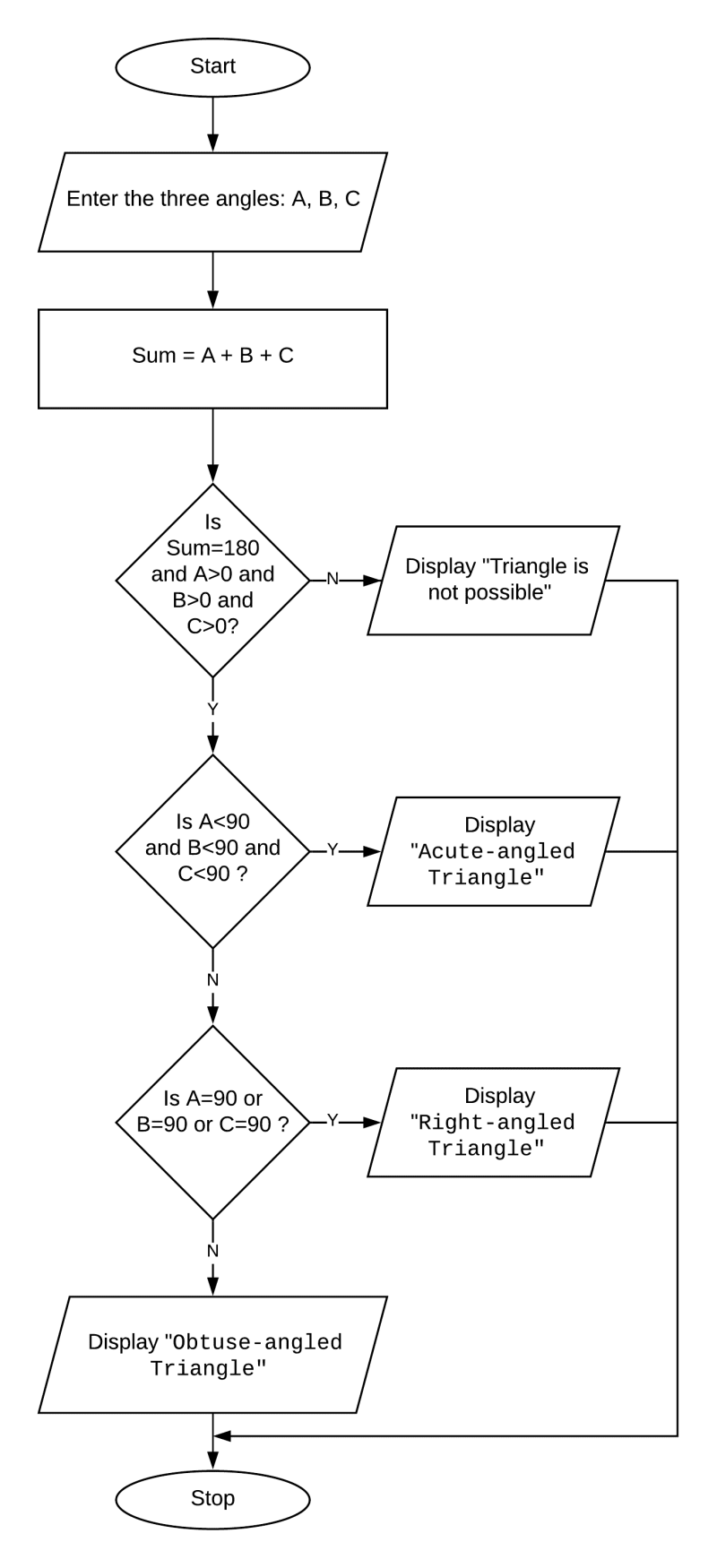#### Question 4

Accept the name and marks obtained by a student in Computer Project. Display the grades as per the table given below:

80% or moreA
60% or more but less than 80%B
40% or more but less than 60%C

Flowchart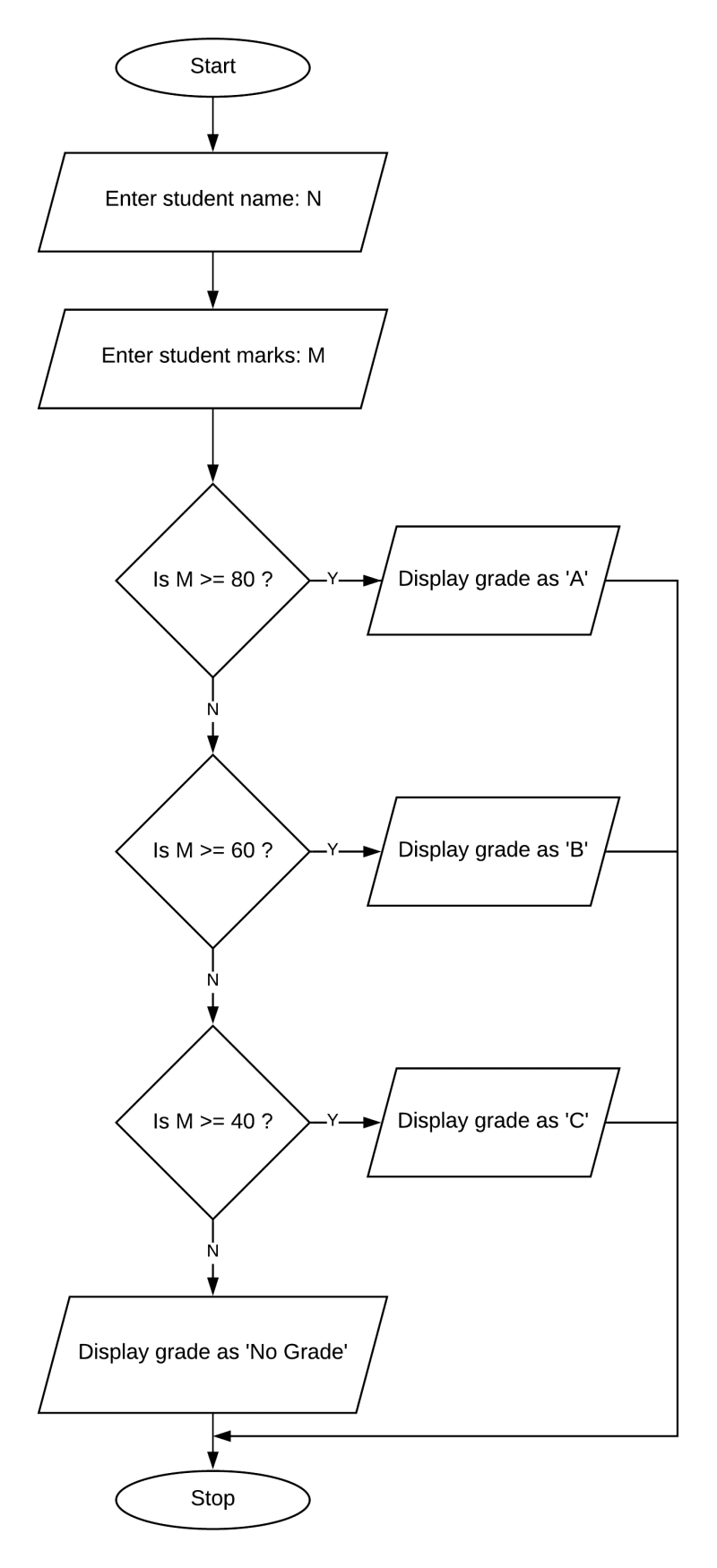#### Question 5

Input the quantity purchased and the rate. Calculate the total purchase price and display it along with the gift to be presented. The gifts to the customers are given as under:

100 and above but less than 500A key ring
500 and above but less than 1000A leather purse
1000 and aboveA pocket calculator

The flowchart will end with a 'Thank you' message.

Flowchart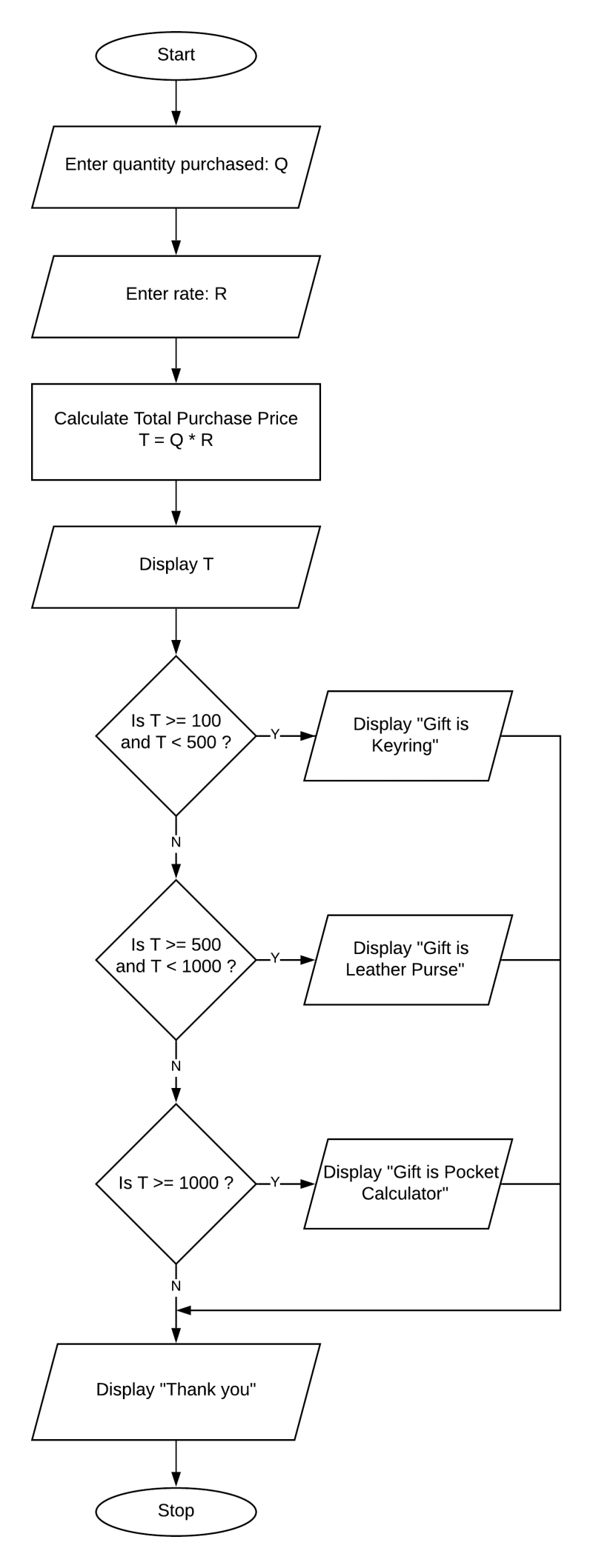#### Question 6

A librarian charges a fine for books, if returned late. The tariff for the fine is given below:

No. of daysFine
For the first ten days40 paise per day
Eleven to twenty days60 paise per day
More than twenty days80 paise per day

Draw a flowchart to calculate the fine assuming that a book is returned 'N' days late.

Flowchart